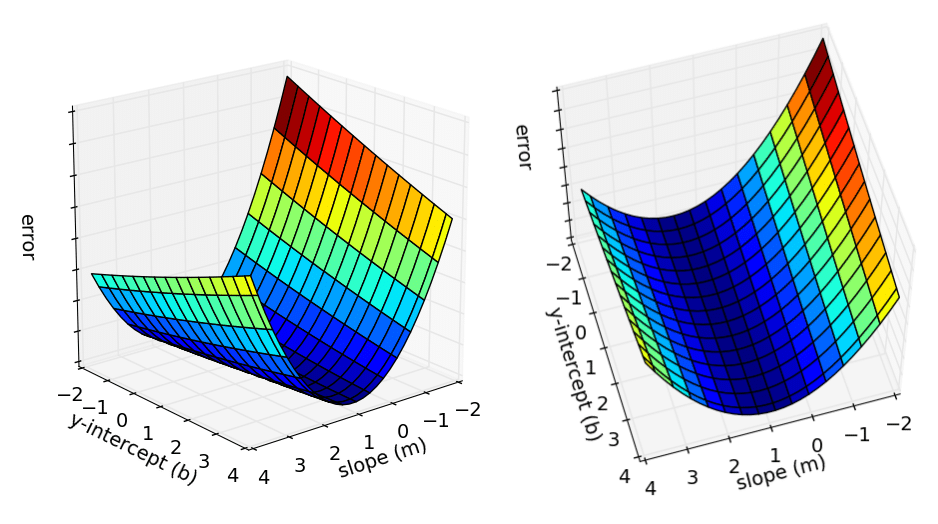# Introduction to Linear Regression

## October 20, 2018

In statistics, linear regression is a linear approach to modelling the relationship between a scalar response and one or more explanatory variables. The case of one explanatory variable is called simple linear regression. For more than one explanatory variable, the process is called multiple linear regression. Put simply, linear regression is all about finding the optimal values of m and c in y = mx + c.

In this example, we will be using linear regression to draw the line of best fit to measure the relationship between student test scores and the number of hours studied. Our only dependency would be NumPy, and gradient descent will be our optimization strategy for linear regression.

# Methodology

Like all machine learning algorithms, we first need to obtain our data to train on. The data file, data.csv contains 100 rows of student test scores and the number of hours studied. Now, let’s start by defining our main function and import everything from Numpy.

The run function will contain our initial values, hyperparameters and function calls. Let’s define our run function.

We use genfromtext to load data from the data.csv file and since the data is in a csv format, we use the comma as a delimiter. Then we define a hyperparameter, our learning_rate determines how fast our model is able to converge (find the optimal value of m and c). Then, we define the initial values of m and c and we initialize the line as a horizontal line. The num_iterations determines the number of times we run our optimizer and since this is a small data set we should be able to reach convergence with just a 1000 iterations. The gradient_descent_runner runs the gradient descent optimizer for the number of iterations specified, in our case for 1000 iterations. Then we compute the error and print out the final, optimized values. Now we need to define the functions that we are calling.

Before we proceed onto defining the step_gradient function which is our optimizer, we need understand the process of gradient descent. Since we are starting off with a horizontal line, we need a way to adjust the m and c parameters so that it will produce the line of best fit for our data. To do this, we first need to know how far off the mark our line is from the data points at a specific x value and we compute this error value using what’s known as the sum of squared errors. The equation for the sum of squared errors as follows:

$\text {Error}_{(m, c)}=\frac{1}{N} \sum_{i=1}^{N}\left(y_{i}-\left(m x_{i}+c\right)\right)^{2}$

Essentially, we subtract the y value of our line from the y value of the data point at a specific x value to obtain the margin of error. We square the error because we only want positive values when we sum and also because we are only interested in the magnitude of the value and not the value itself. Then we sum the error across all points and divide them by the number of points to obtain the total error. Now that we have calculated the total error, our goal would be to minimize this error value. Before we look at how we can minimize the error value, let’s look at this excellent visualization.Relationship between the error, y-intercept (b) and the slope (m)

The visualization shows all the possible values of m, c (shown as b in the visualization) and error. We know that our goal is to adjust the m and c values to obtain the lowest possible error value. The lowest possible error value is at the bottom of the curve, in the dark blue region of the graph. This region is known as the local minima. The bottom of the curve has a gradient of 0 and if we were to find the gradient of the curve at specific m and c values and adjust the m and c values such that the gradient eventually reaches 0, we will be able to achieve convergence easily. To do this, we need to find the gradient of m and c. The gradients of m and c are partial derivatives and they can be computed using the following equations.

$\frac{\partial}{\partial c}=\frac{2}{N} \sum_{i=1}^{N}-\left(y_{i}-\left(m x_{i}+c\right)\right)$ $\frac{\partial}{\partial m}=\frac{2}{N} \sum_{i=1}^{N}-x_{i}\left(y_{i}-\left(m x_{i}+c\right)\right)$

Let’s translate the above equation into code.

The initial gradient of c and m is 0 and the number of points is defined as N. We then calculate the gradient using the above formulas and update the current value of c and m. We update the values of c and m by subtracting the multiplication of the learning rate and the gradient values. If our gradient is positive that means that increasing the value of m or c would cause the error to increase and if the gradient is negative, increasing the value of m or c would cause a decrease in the error value. So if we subtract the gradient of m or c from the current value of m or c this would cause the value of m or c to increase if the gradient is negative and decrease if the gradient is positive. We multiply the gradient by the learning rate so that we can achieve convergence at a slow and steady pace. Computing the error value of the line of best fit would be useful for us to see how good or bad our line is at measuring the relationship between student test scores and the number of hours studied. We compute the error value using the following function.

Running the code,